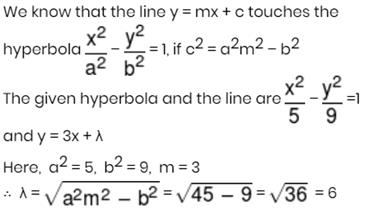Courses

# Mathematics Test 2 - Co-Ordinate Geometry, Sequence And Series, Relation Functions,Trigonometry

## 25 Questions MCQ Test Mock Test Series for JEE Main & Advanced 2021 | Mathematics Test 2 - Co-Ordinate Geometry, Sequence And Series, Relation Functions,Trigonometry

Description
This mock test of Mathematics Test 2 - Co-Ordinate Geometry, Sequence And Series, Relation Functions,Trigonometry for JEE helps you for every JEE entrance exam. This contains 25 Multiple Choice Questions for JEE Mathematics Test 2 - Co-Ordinate Geometry, Sequence And Series, Relation Functions,Trigonometry (mcq) to study with solutions a complete question bank. The solved questions answers in this Mathematics Test 2 - Co-Ordinate Geometry, Sequence And Series, Relation Functions,Trigonometry quiz give you a good mix of easy questions and tough questions. JEE students definitely take this Mathematics Test 2 - Co-Ordinate Geometry, Sequence And Series, Relation Functions,Trigonometry exercise for a better result in the exam. You can find other Mathematics Test 2 - Co-Ordinate Geometry, Sequence And Series, Relation Functions,Trigonometry extra questions, long questions & short questions for JEE on EduRev as well by searching above.
QUESTION: 1

### Mid-points of the sides AB and AC of a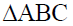are (3,5) and (–3,–3) respectively, then the length of the side BC is

Solution: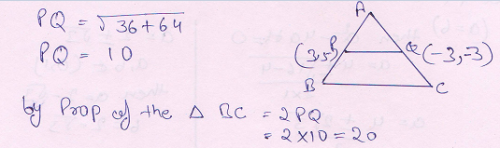QUESTION: 2

### The co-ordinates of the middle points of the sides of a triangle are (4,2),(3,3) and (2,2), then the coordinates of its centroid are

Solution: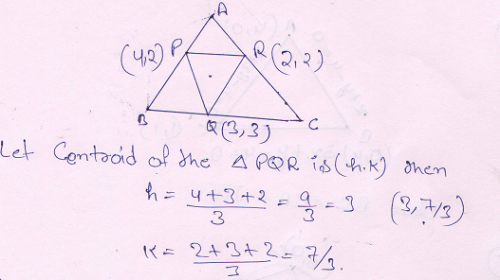QUESTION: 3

### If a and b are real numbers between 0 and 1 such that the points (a,1),(1,b) and (0,0) form an equilateral triangle, then a, b are

Solution: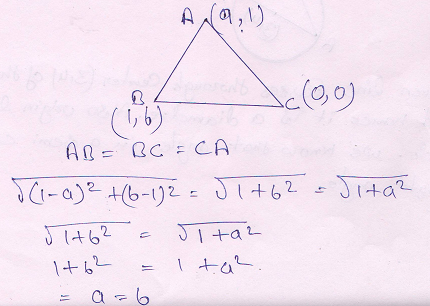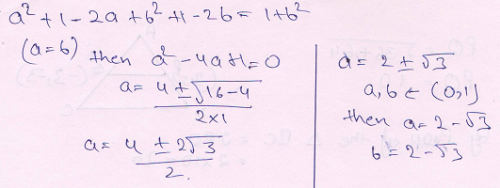QUESTION: 4
The straight lines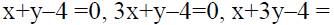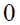form a triangle which is
Solution: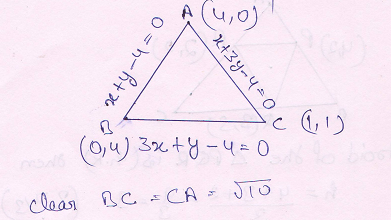QUESTION: 5
The straight lines joining the origin to the points of intersection of the line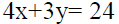with the circle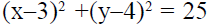are
Solution: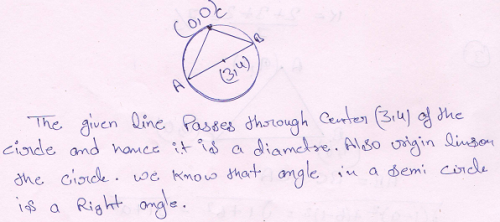QUESTION: 6
The area of circle centred at (1,2) and passing through (4,6) is
Solution: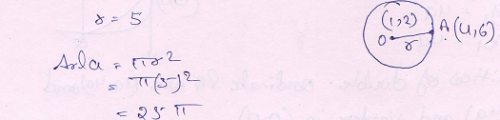QUESTION: 7
The equation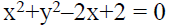represents
Solution: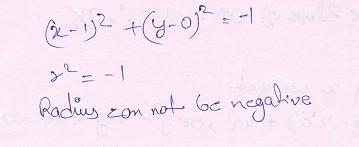QUESTION: 8
A diameter of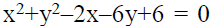is a chord to circle centre (2,1), then radius of the circle is
Solution: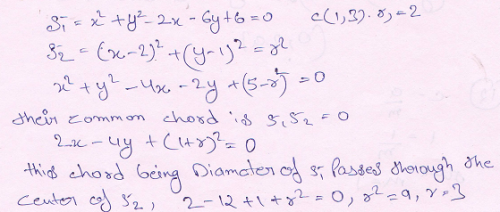QUESTION: 9
In the parabola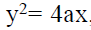, the length of the chord passing through the vertex and inclined to the axis at an angle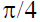is
Solution: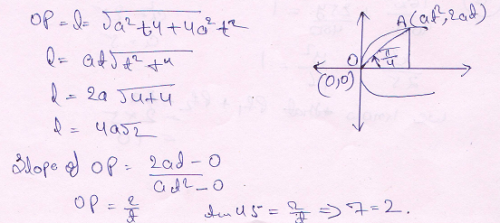QUESTION: 10
The angle made by a double ordinate of length 8a at the vertex of the parabola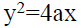is
Solution: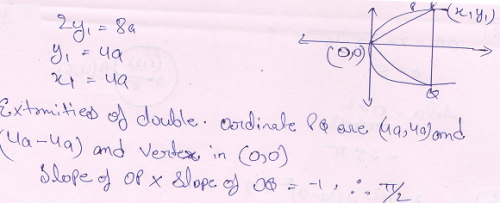QUESTION: 11
The straight line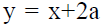touches the parabola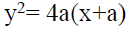at the point
Solution: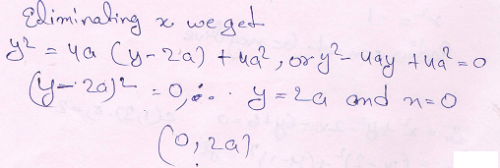QUESTION: 12

The line y = mx + 1 is a tangent to the parabola y2 = 4ax, if

Solution: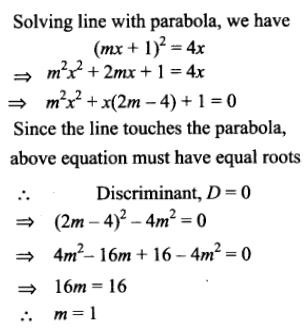QUESTION: 13
If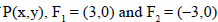and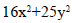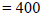, then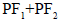equals

Solution: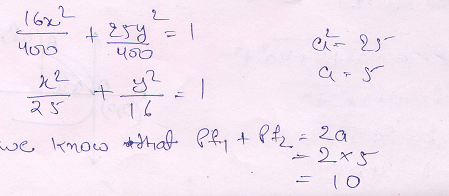QUESTION: 14

An ellipse has OB as a semi minor axis. F,F` are its foci, and the angle FBF` is a right angle. Then the eccentricity of the ellipse is

Solution: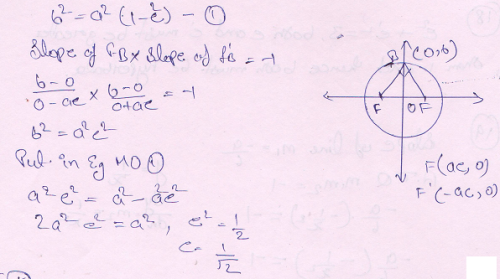QUESTION: 15

If the straight line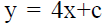is a tangent to the ellipse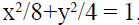, then c will be equal to

Solution: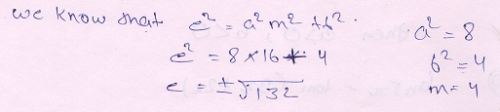QUESTION: 16
The eccentricity of the hyperbola with latus rectum 12 and semi-conjugate axis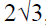, is
Solution: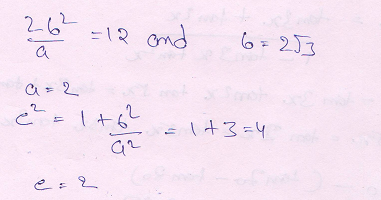QUESTION: 17
If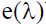be the eccentricity of rectangular hyperbola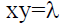then the value of e(1)–e(6) is
Solution: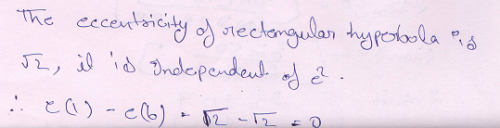QUESTION: 18
If e and e` be the eccentricities of two conics S and S` such that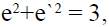then both S and S` are
Solution: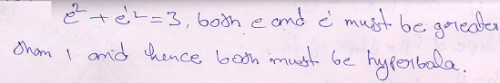QUESTION: 19
If the line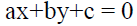is normal to xy=1, then
Solution: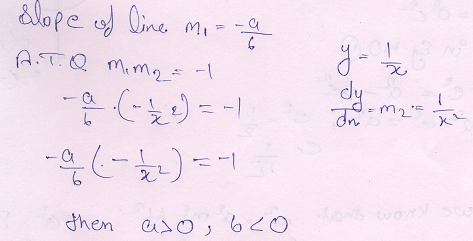QUESTION: 20
tan5x–tan 3x–tan2x =
Solution: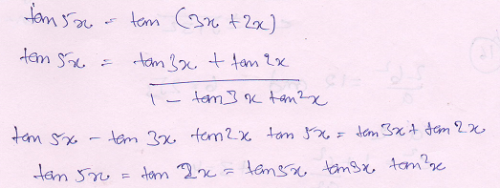*Answer can only contain numeric values
QUESTION: 21

The value of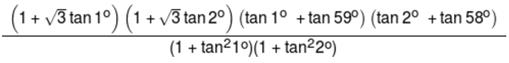is:-

Solution: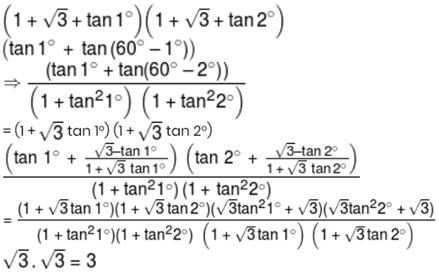*Answer can only contain numeric values
QUESTION: 22

Find number of surjection from A to B where
A= {1, 2, 3, 4}, B = {a, b}:

Solution:
*Answer can only contain numeric values
QUESTION: 23

Sum of all the solution of equation sinπx + cosπx= 0 in x ∈ [0, 100]

Solution: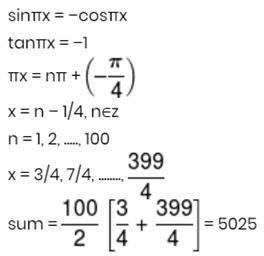*Answer can only contain numeric values
QUESTION: 24

If a,b,c are in A.P. and one root of ax2 + bx + c = 0 is 2 then other root is?

Solution: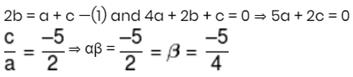*Answer can only contain numeric values
QUESTION: 25

If the line y = 3x + λ touches the hyperbola 9x2 – 5y2 = 45, then the value of λ is

Solution: